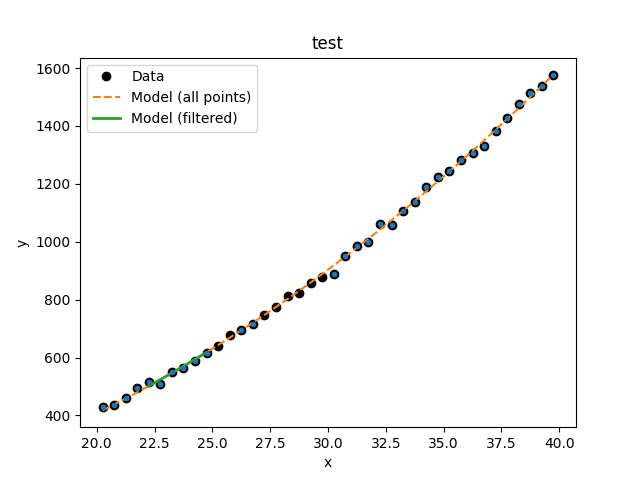# What data is to be fit?

The Sherpa `Data` class is used to carry around the data to be fit: this includes the independent axis (or axes), the dependent axis (the data), and any necessary metadata. Although the design of Sherpa supports multiple-dimensional data sets, the current classes only support one- and two-dimensional data sets.

The following modules are assumed to have been imported:

```>>> import numpy as np
>>> import matplotlib.pyplot as plt
>>> from sherpa.stats import LeastSq
>>> from sherpa.optmethods import LevMar
>>> from sherpa import data
```

## Names

The first argument to any of the Data classes is the name of the data set. This is used for display purposes only, and can be useful to identify which data set is in use. It is stored in the `name` attribute of the object, and can be changed at any time.

## The independent axis

The independent axis - or axes - of a data set define the grid over which the model is to be evaluated. It is referred to as `x`, `x0`, `x1`, … depending on the dimensionality of the data (for binned datasets there are `lo` and `hi` variants).

Although dense multi-dimensional data sets can be stored as arrays with dimensionality greater than one, the internal representation used by Sherpa is often a flattened - i.e. one-dimensional - version.

The `sherpa.astro.data.DataPHA` class can be thought of as being either unbinned or binned, depending on the units (channels or energy/wavelength), and this is discussed in the PHA example page.

## The dependent axis

This refers to the data being fit, and is referred to as `y`.

### Unbinned data

Unbinned data sets - defined by classes which do not end in the name `Int` - represent point values; that is, the the data value is the value at the coordinates given by the independent axis. Examples of unbinned data classes are `Data1D` and `Data2D`.

```>>> np.random.seed(0)
>>> x = np.arange(20.25, 40, 0.5)
>>> y = x**2 + np.random.normal(0, 10, size=x.size)
>>> d1 = data.Data1D('test', x, y)
>>> print(d1)
name      = test
x         = Float64
y         = Float64
staterror = None
syserror  = None
>>> print(d1.x)
[20.25 20.75 21.25 21.75 22.25 22.75 23.25 23.75 24.25 24.75 25.25 25.75
26.25 26.75 27.25 27.75 28.25 28.75 29.25 29.75 30.25 30.75 31.25 31.75
32.25 32.75 33.25 33.75 34.25 34.75 35.25 35.75 36.25 36.75 37.25 37.75
38.25 38.75 39.25 39.75]
>>> print(d1.y)
[ 427.70302346  434.56407208  461.34987984  495.47143199  513.7380799
507.7897212   550.06338418  562.54892792  587.03031148  616.66848502
639.00293571  677.60523507  696.67287725  716.77925016  747.00113233
773.39924327  813.00329073  824.51091736  858.69317702  876.52154261
889.53260184  952.09868595  985.20686199 1000.6408498  1062.76004624
1058.01884325 1106.02008517 1137.1906615  1188.39029214 1222.2560877
1244.11197426 1281.8441252  1305.18464252 1330.75453532 1384.08337851
1426.62598969 1475.36540681 1513.58629849 1536.68923183 1577.03947249]
>>> plt.plot(d.x, d.y, 'o')
```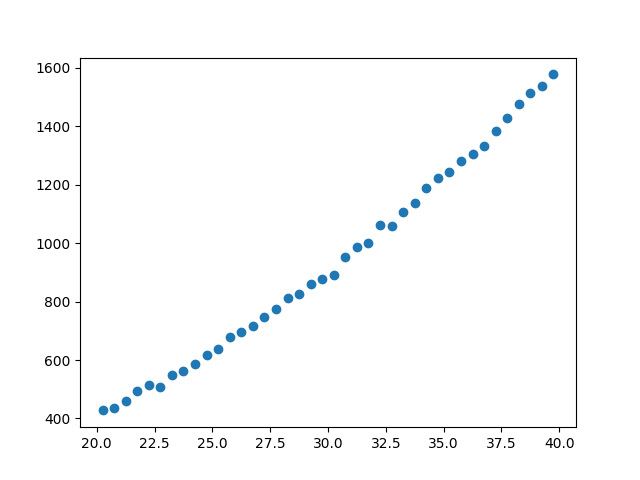### Binned data

Binned data sets represent values that are defined over a range, such as a histogram. The integrated model classes end in `Int`: examples are `Data1DInt` and `Data2DInt`.

It can be a useful optimisation to treat a binned data set as an unbinned one, since it avoids having to estimate the integral of the model over each bin. It depends in part on how the bin size compares to the scale over which the model changes.

```>>> z = np.random.gamma(20, scale=0.5, size=1000)
>>> (y, edges) = np.histogram(z)
>>> d2 = data.Data1DInt('gamma', edges[:-1], edges[1:], y)
>>> print(d2)
name      = gamma
xlo       = Float64
xhi       = Float64
y         = Int64
staterror = None
syserror  = None
>>> plt.clf()
>>> plt.bar(d2.xlo, d2.y, d2.xhi - d2.xlo, align='edge')
```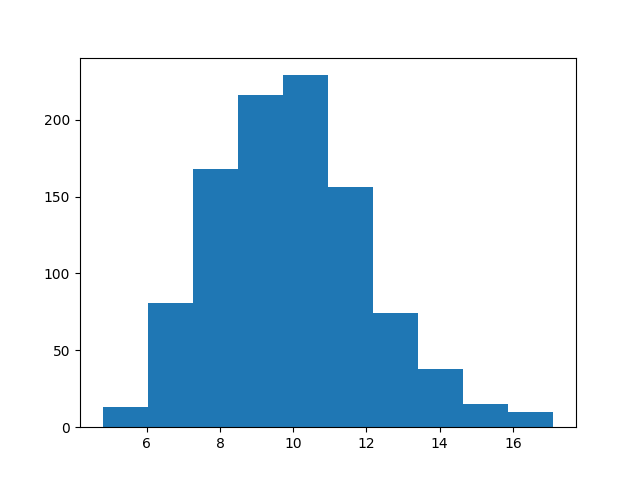## Errors

There is support for both statistical and systematic errors by either using the `staterror` and `syserror` parameters when creating the data object, or by changing the `staterror` and `syserror` attributes of the object.

## Filtering data

Sherpa supports filtering data sets; that is, temporarily removing parts of the data (perhaps because there are problems, or to help restrict parameter values). There are routines to filter the data and to find out what bins have been selected.

The `ignore()` and `notice()` methods are used to define the ranges to exclude or include. The `mask` attribute indicates whether a filter has been applied: if it returns `True` then no filter is set, `False` when all data has been filtered out, otherwise it is a bool array where `False` values indicate those elements that are to be ignored. Note that the Sherpa definition is opposite of the convention in numpy, where `True` indicates a masked value to be ignored, while Sherpa uses `False` for this purpose. For example, the following hides those values where the independent axis values are between 21.2 and 22.8:

```>>> d1.ignore(21.2, 22.8)
array([21.25, 21.75, 22.25, 22.75])
```

Note

The meaning of the range selected by the `notice` and `ignore` calls depends on the data class: `sherpa.data.Data1DInt` and `sherpa.astro.data.DataPHA` classes can treat the upper limit differently (it can be inclusive or exclusive) and 2D datasets such as `sherpa.data.Data2D` can use filters that use both axes.

The `get_filter()` method will report a string representation of the existing filter, so here showing that the bins between 21.2 and 22.8 have been ignored:

```>>> print(d1.get_fiter())
20.2500:20.7500,23.2500:39.7500
```

Note

The filter does not record the requested changes - that is here the 21.2 to 22.8 arguments to `ignore()` - but instead reflects the selected bins in the data set.

After this, a fit to the data will ignore these values, as shown below, where the number of degrees of freedom of the first fit, which uses the filtered data, is three less than the fit to the full data set (the call to `notice()` removes the filter since no arguments were given):

```>>> from sherpa.models import Polynom1D
>>> from sherpa.fit import Fit
>>> mdl = Polynom1D()
>>> mdl.c2.thaw()
>>> fit = Fit(d1, mdl, stat=LeastSq(), method=LevMar())
>>> res1 = fit.fit()

>>> d1.notice()
>>> res2 = fit.fit()

>>> print(f"Degrees of freedom: {res1.dof} vs {res2.dof}")
Degrees of freedom: 34 vs 38
```

### Resetting the filter

The `notice()` method can be used to reset the filter - that is, remove all filters - by calling with no arguments (or, equivalently, with two `None` arguments). Similarly the `ignore()` method called with no arguments will remove all points.

### The first filter call

When a data set is created no filter is applied, which is treated as a special case (this also holds if the filters have been reset). The first call to `notice()` will restrict the data to just the requested range, and subsequent calls will add the new data. So with no filter we see the whole data range:

```>>> d1.notice()
>>> print(d1.get_fiter(format='%.2f'))
20.25:39.75
```

The first `notice()` call restricts to just this range:

```>>> d1.notice(25, 27)
>>> print(d1.get_fiter(format='%.1f'))
25.25:26.75
```

Subsequent `notice()` calls add to the selected range:

```>>> d1.notice(30, 35)
>>> print(d1.get_fiter(format='%.1f'))
25.25:26.75,30.25:34.75
```

### The edges of a filter

Mathematically the two sets of commands below should select the same range, but it can behave slightly different for values at the edge of the filter (or within the edge bins for `Data1DInt` and `DataPHA` objects):

```>>> d1.notice(25, 35)
>>> d1.ignore(29, 31)
```
```>>> d1.notice(25, 29)
>>> d1.notice(31, 35)
```

### Accessing filtered data

Although the `mask` attribure can be used to manually filter the data, many data accessors accept a `filter` argument which, if set, will filter the requested data. When a filter is applied, `get_x()` and `get_y()` will default to returning all the data but if the `filter` argument is set then the current filter will be applied:

```>>> d1.notice()
>>> d1.notice(21.1, 23.5)
>>> d1.get_x()
[20.25 20.75 21.25 21.75 22.25 22.75 23.25 23.75 24.25 24.75 25.25 25.75
26.25 26.75 27.25 27.75 28.25 28.75 29.25 29.75 30.25 30.75 31.25 31.75
32.25 32.75 33.25 33.75 34.25 34.75 35.25 35.75 36.25 36.75 37.25 37.75
38.25 38.75 39.25 39.75]
>>> d1.get_y()
[ 427.70302346  434.56407208  461.34987984  495.47143199  513.7380799
507.7897212   550.06338418  562.54892792  587.03031148  616.66848502
639.00293571  677.60523507  696.67287725  716.77925016  747.00113233
773.39924327  813.00329073  824.51091736  858.69317702  876.52154261
889.53260184  952.09868595  985.20686199 1000.6408498  1062.76004624
1058.01884325 1106.02008517 1137.1906615  1188.39029214 1222.2560877
1244.11197426 1281.8441252  1305.18464252 1330.75453532 1384.08337851
1426.62598969 1475.36540681 1513.58629849 1536.68923183 1577.03947249]
>>> d1.get_x(filter=True)
[21.25 21.75 22.25 22.75 23.25]
>>> d1.get_y(filter=True)
[461.34987984 495.47143199 513.7380799  507.7897212  550.06338418]
```

### Enhanced filtering

Certain data classes - in particular `sherpa.astro.data.DataIMG` and `sherpa.astro.data.DataPHA` - extend and enhance the filtering capabilities to:

## Visualizing a data set

The data objects contain several methods which can be used to visualize the data, but do not provide any direct plotting or display capabilities. There are low-level routines which provide access to the data - these include the `to_plot()` and `to_contour()` methods - but the preferred approach is to use the classes defined in the `sherpa.plot` module, which are described in the visualization section:

```>>> from sherpa.plot import DataHistogramPlot
>>> pdata = DataHistogramPlot()
>>> pdata.prepare(d2)
>>> pdata.plot()
```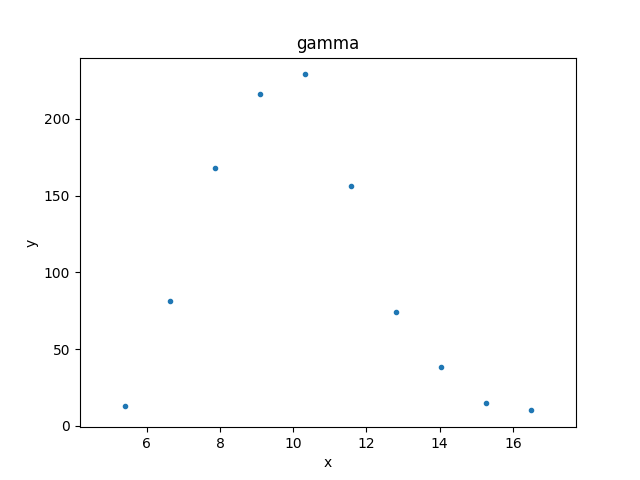Although the data represented by `d2` is a histogram, the values are displayed at the center of the bin.

Plot preferences can be changed in the `plot` call:

```>>> pdata.plot(linestyle='solid', marker='')
```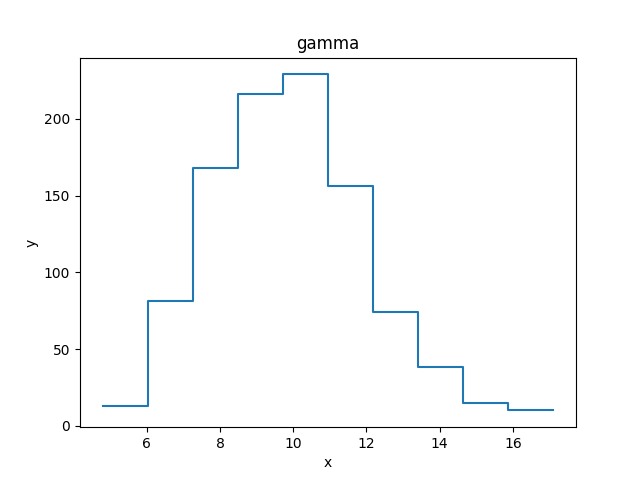Note

The supported options depend on the plot backend (although at present only matplotlib is supported).

The plot objects automatically handle any filters applied to the data, as shown below.

```>>> from sherpa.plot import DataPlot
>>> pdata = DataPlot()
>>> d1.notice()
>>> d1.ignore(25, 30)
>>> d1.notice(26, 27)
>>> pdata.prepare(d1)
>>> pdata.plot()
```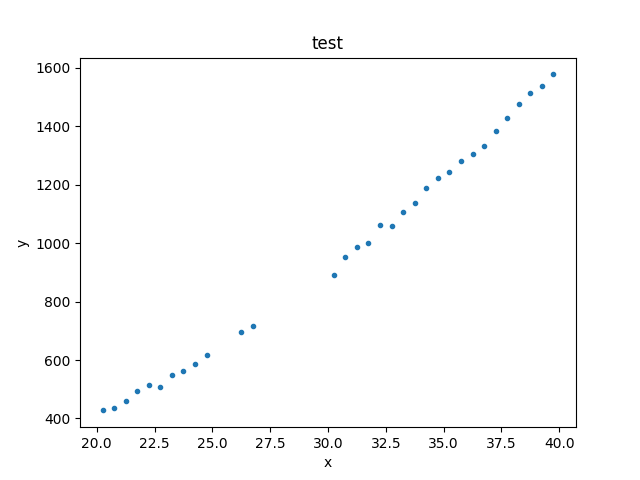Note

The plot object stores the data given in the `prepare()` call, so that changes to the underlying objects will not be reflected in future calls to `plot()` unless a new call to `prepare()` is made.

```>>> d1.notice()
```

At this point, a call to `pdata.plot()` would re-create the previous plot, even though the filter has been removed from the underlying data object.

## Evaluating a model

The `eval_model()` and `eval_model_to_fit()` methods can be used to evaluate a model on the grid defined by the data set. The first version uses the full grid, whereas the second respects any filtering applied to the data.

```>>> d1.notice(22, 25)
>>> y1 = d1.eval_model(mdl)
>>> y2 = d1.eval_model_to_fit(mdl)